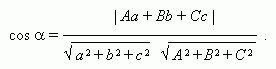Straight line

Equation of straight line going through two different points.

Parametric equation of straight line.

Equation of intersection line of two planes.

Parallelism condition of straight lines.

Perpendicularity condition of straight lines.

Angle between straight lines.

Angle between straight line and plane.

An equation of straight line going through two different points ( õ 1 , ó 1 , z 1 )  and ( õ 2 , ó 2 , z 2 ):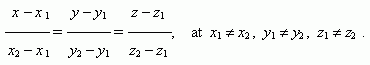A parametric equation of straight line , going through a point ( õ 0 , ó 0 , z 0 ) and parallel to a direction vector ( a , b , ñ ) of a straight line: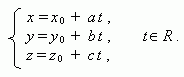Let two planes Àõ + Âó + Ñ z+ D = 0 and E õ + F ó + Gz+ H = 0 be given, moreover, their normal vectors aren't collinear, then the set of simultaneous equations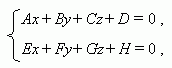describes an intersection line of these planes.

Let ( a , b , ñ ) and  ( p , q , r ) be direction vectors of two straight lines, then a parallelism condition of straight lines is :

aq bp = br cq = ar cp = 0 ,

a perpendicularity condition of straight lines is :

ap + bq + cr = 0 ,

an angle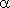between straight lines: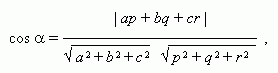an anglebetween a straight line and a plane: